# Algebra 1 : How to multiply monomial quotients

## Example Questions

1 2 3 4 6 Next →

### Example Question #581 : Variables

Simplify the expression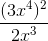.

What is the coefficient in this expression after it is simplified?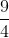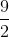Explanation:

To simplify the expression use the rule of exponents which states that when like bases are raised to a power the exponents are multiplied together. When like bases are divided that means that their exponents are subtracted.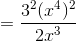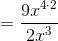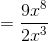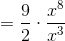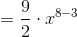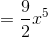The coefficient of this simplified form is.

### Example Question #582 : Variables

Simplify: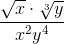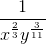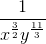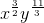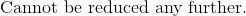Explanation:

In order to simplify, rewrite the numerator in terms of exponential powers.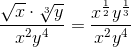Because similar bases are divided, subtract the powers of the numerator with the denominator.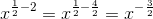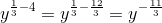Since these two variables have negative exponents, we can simply take the inverse of both to eliminate the negative exponents.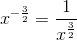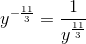Multiply both fractions.

The answer is:1 2 3 4 6 Next →